### IMO Shortlist 2010 problem C4

Kvaliteta:
Avg: 0,0
Težina:
Avg: 7,0
Each of the six boxes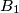$B_1$,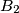$B_2$,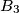$B_3$,$B_4$,$B_5$,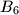$B_6$ initially contains one coin. The following operations are allowed

Type 1) Choose a non-empty box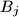$B_j$,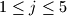$1\leq j \leq 5$, remove one coin from$B_j$ and add two coins to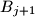$B_{j+1}$;

Type 2) Choose a non-empty box$B_k$,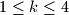$1\leq k \leq 4$, remove one coin from$B_k$ and swap the contents (maybe empty) of the boxes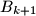$B_{k+1}$ and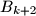$B_{k+2}$.

Determine if there exists a finite sequence of operations of the allowed types, such that the five boxes$B_1$,$B_2$,$B_3$,$B_4$,$B_5$ become empty, while box$B_6$ contains exactly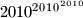$2010^{2010^{2010}}$ coins.

Proposed by Hans Zantema, Netherlands
Izvor: Međunarodna matematička olimpijada, shortlist 2010